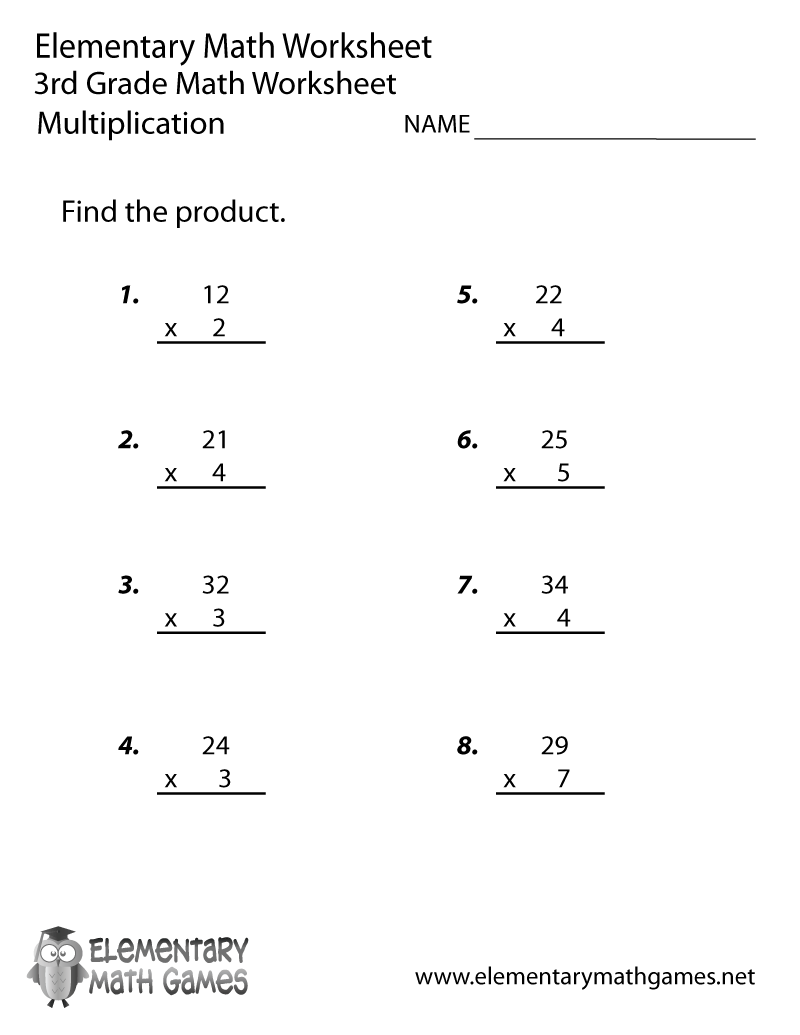## Learn and Practice Multiplication

This third grade math worksheet will help students who are learning multiplication. There are 8 math problems for third graders to solve and plenty of space for them to work out the math.

Here are the two versions of this free third grade math worksheet: